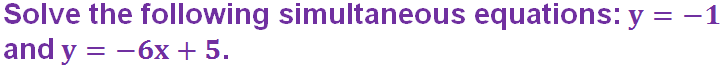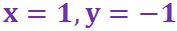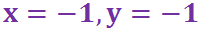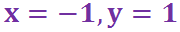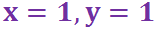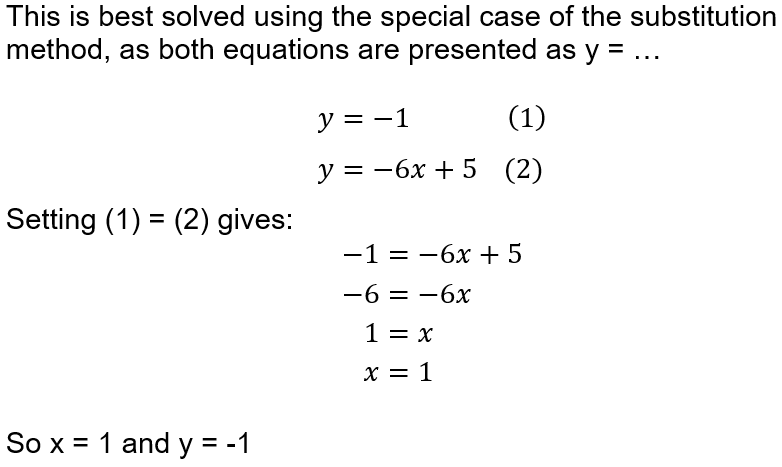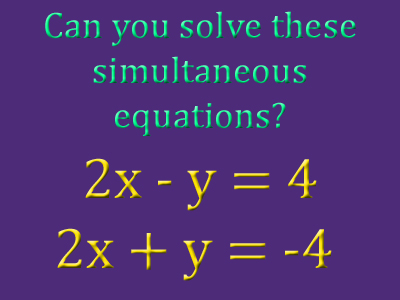If you can then you already have question 4 completed!

# Simultaneous Equations (F)

Simultaneous equations are a system of 2 equations, with 2 variables (i.e. letters) that have to be solved at the same time, or simultaneously. Whilst they might sound a bit scary, once you learn the process and apply common sense to your workings you will have them sorted in no time. See how well you get on in this GCSE Maths quiz!

You should be able to recognise a problem that is based on simultaneous equations – it will have two equations, and include two different variables. Once you spot this you need to decide which method will be the easier to use.

Communication is an important part of solving simultaneous equations, so you should start by writing down and labelling each equation – if using numbers, put a big circle around each one so it doesn’t get swallowed up into the equation! Then decide if substitution or elimination is the better method. Substitution is best when a variable is the subject of one of the equations, so it will start with y = …. or x = ….. Substitute that expression into the second equation. This will produce one equation with one unknown, which can be solved. Substitute this value back into the first equation to find the second variable.

The elimination method works by combining the two equations to get rid, or eliminate, one of the variables. Look to see if there are equal amounts of one of the variables in both equations (ignore the sign at this stage) – this is the variable you will eliminate. For example, if one equation includes ‘+2x’ and the second equation has ‘-2x’ then you can use this method. You combine the equations by adding or subtracting them – if the SIGNS (of the variable you are eliminating) are the SAME you SUBTRACT. Just as in substitution, this will produce one equation with one unknown, which can be solved. Substitute this value back into either equation to find the value of the second variable.

Question 1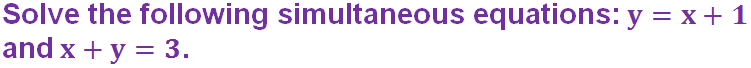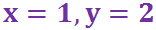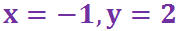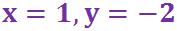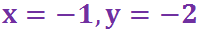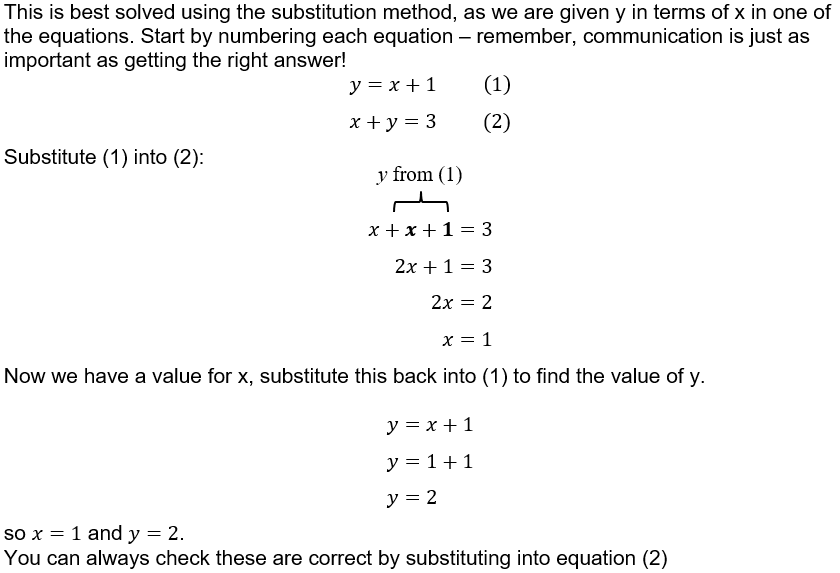Question 2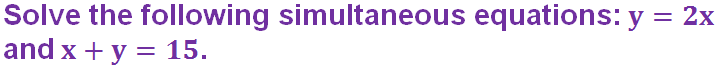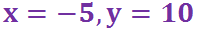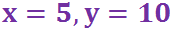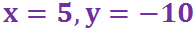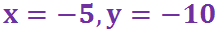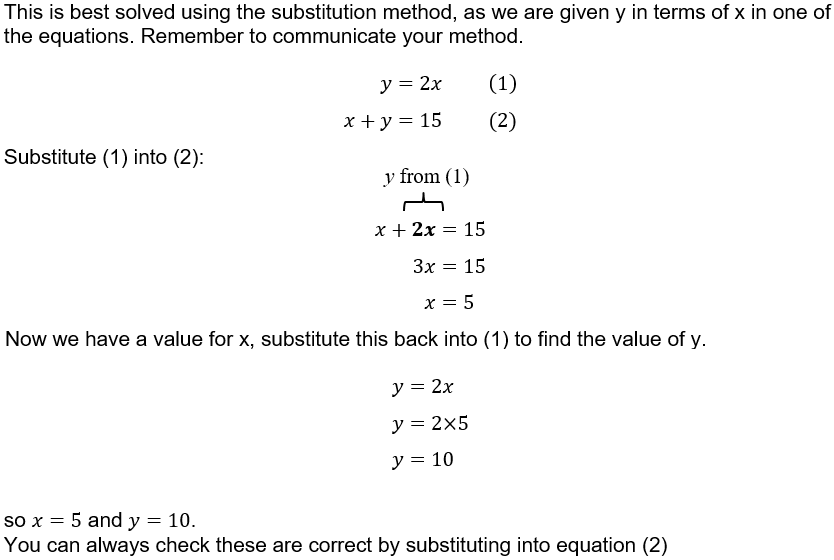Question 3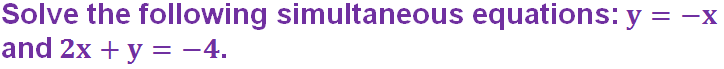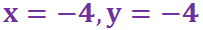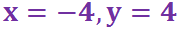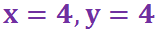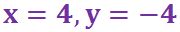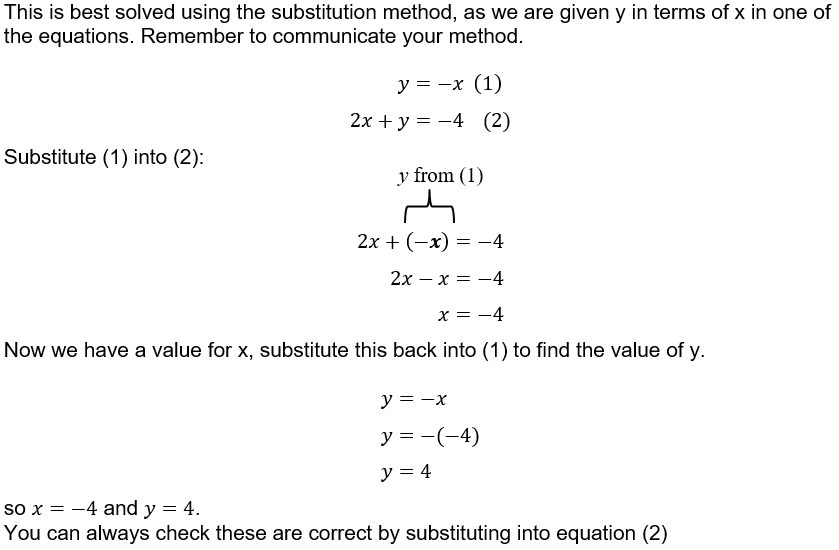Question 4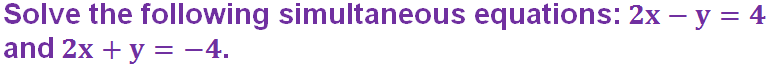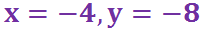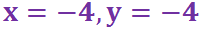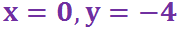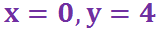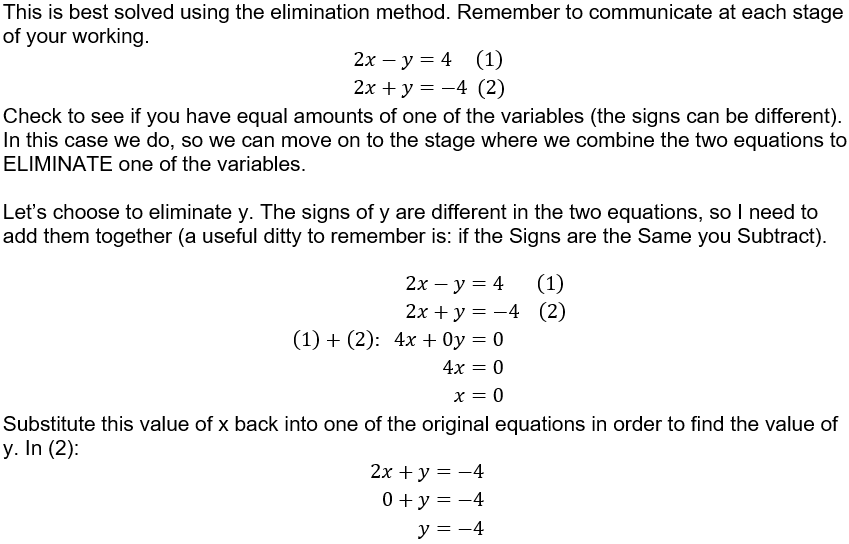Question 5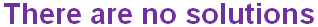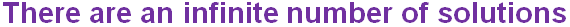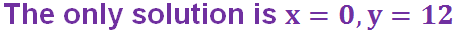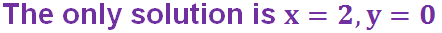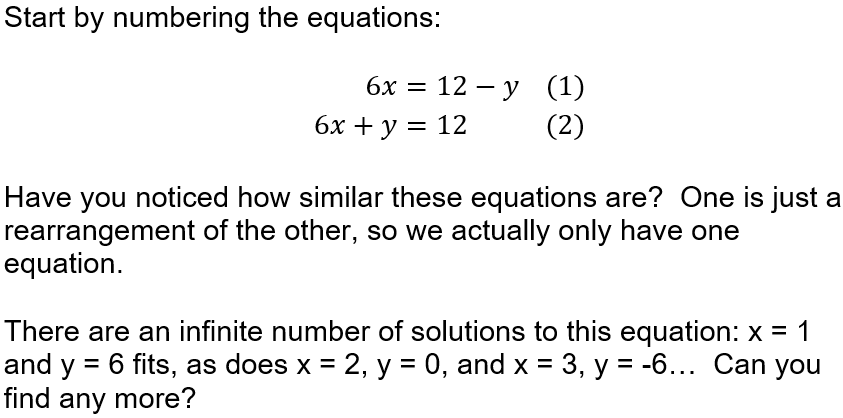Question 6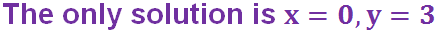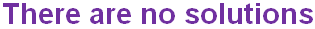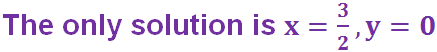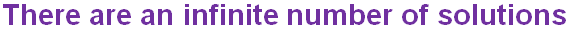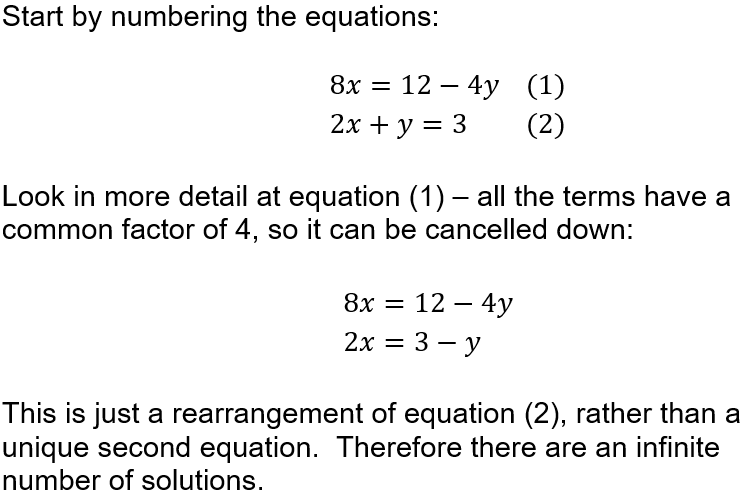Question 7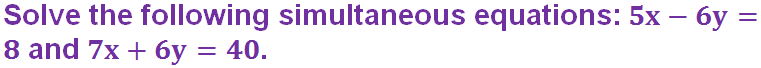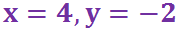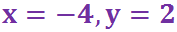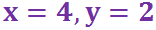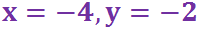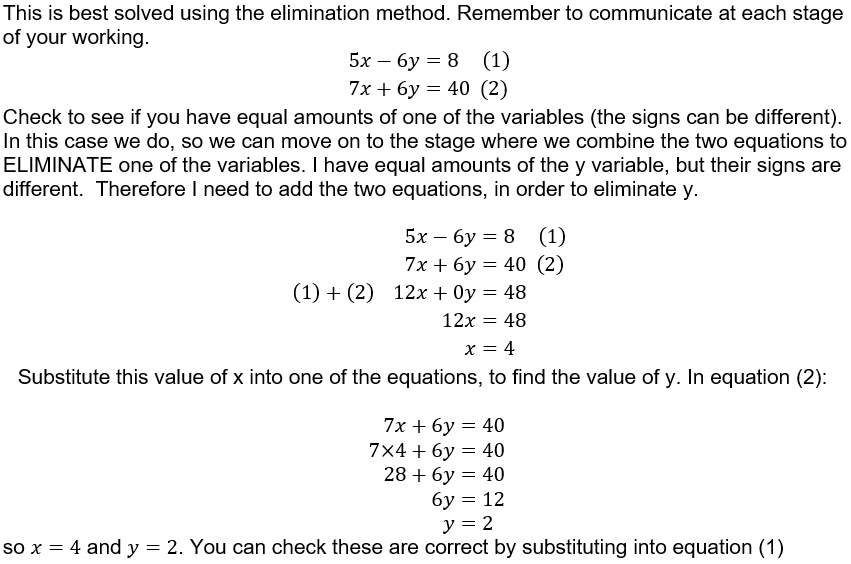Question 8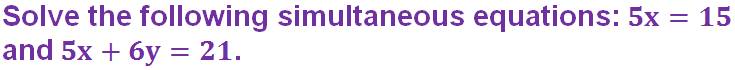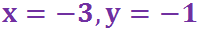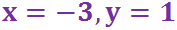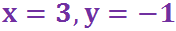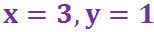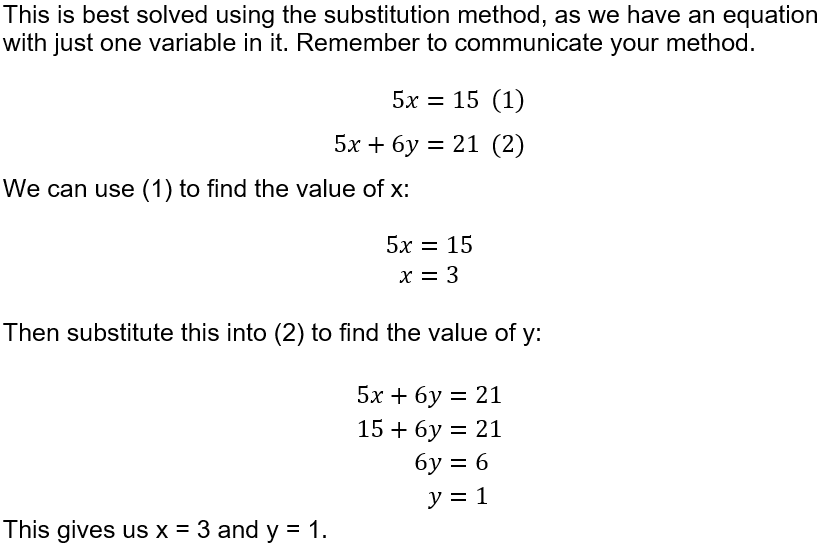Question 9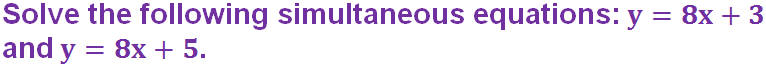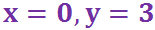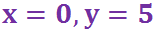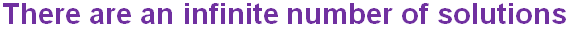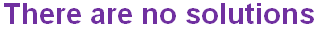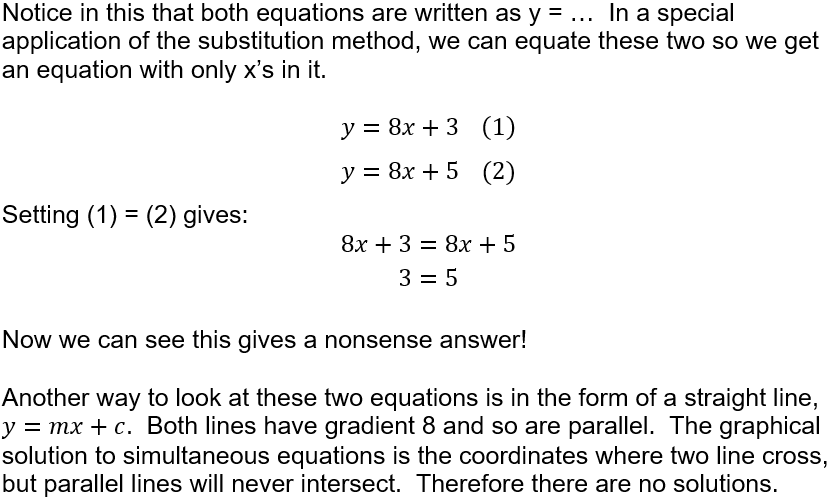Question 10